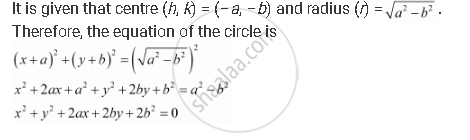CBSE (Arts) Class 11CBSE
Share
Notifications

View all notifications
Books Shortlist
Your shortlist is empty

# Find the Equation of the Circle with Centre (–A, –B) and Radius Sqrt(A^2-b^2) - CBSE (Arts) Class 11 - Mathematics

Login
Create free account

Forgot password?

#### Question

Find the equation of the circle with centre (–a, –b) and radius sqrt(a^2-b^2)

#### Solution

The equation of a circle with centre (hk) and radius is given as

(x­ – h)2 + (y ­– k)2 = r2Is there an error in this question or solution?

#### APPEARS IN

Solution Find the Equation of the Circle with Centre (–A, –B) and Radius Sqrt(A^2-b^2) Concept: Concept of Circle.
S